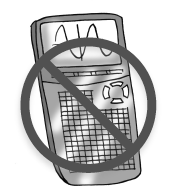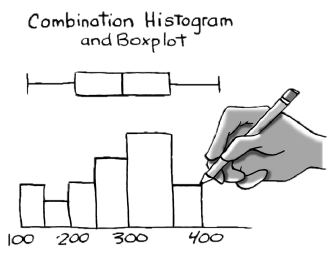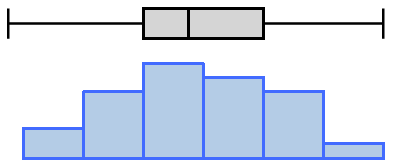### Home > GB8I > Chapter 10 Unit 11 > Lesson INT1: 10.1.2 > Problem10-33

10-33.

The GPAs of a sample of $26$ community college applicants were selected and listed in ascending order below. Do not use a calculator for this problem.

 $1.58$ $1.87$ $2.32$ $2.44$ $2.46$ $2.46$ $2.50$ $2.63$ $2.69$ $2.72$ $2.78$ $2.81$ $2.83$ $2.99$ $3.10$ $3.16$ $3.30$ $3.42$ $3.46$ $3.49$ $3.54$ $3.67$ $3.75$ $3.87$ $3.89$ $4.29$1. Find the five number summary of the GPAs of these applicants.

Remember, a five number summary is the minimum, first quartile, median, third quartile, and maximum. In this set, those would be the $1$st, $7$th, mean of the $13$th and $14$th, $20$th, and $26$th numbers, respectively.

Minimum: $1.58$
First Quartile: $2.50$
Median: $2.91$
Second Quartile: $3.49$
Maximum: $4.29$1. On grid paper, create a combination histogram and boxplot. Use an interval of $0.5$ on the $x$-axis. (A combination histogram and boxplot starts with a histogram. Then place a boxplot on top of the histogram. Help with creating a boxplot is in the Math Notes box for this lesson. Use the same $x$-axis scale for both the histogram and the boxplot.)

An example combination histogram and boxplot has been provided.2. Describe the center, shape, spread, and outliers. To review descriptions of “shape,” see the Math Notes box in the next lesson. To describe “spread” use the IQR, reviewed in the Math Notes box in this lesson.

Where is the center?
Are most of the points concentrated there?
How many peaks are there?
Is the shape symmetric?
Are there any outliers?
What is the IQR?

The center is at about $2.91$ points.
The shape is single-peaked and symmetric with no apparent outliers.